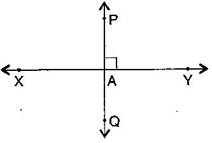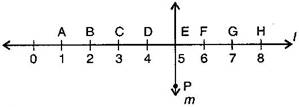1. /
2. CBSE
3. /
4. Class 06
5. /
6. Mathematics
7. /
8. NCERT Solutions for Class...

# NCERT Solutions for Class 6 Maths Exercise 5.5

NCERT solutions for Maths Understanding elementary## NCERT Solutions for Class 6 Maths Understanding elementary

###### Question 1.Which of the following are models for perpendicular lines:

(a)The adjacent edges of a table top.

(b)The lines of a railway track.

(c)The line segments forming the letter ‘L’.

(d)The letter V.

(b) Not perpendicular

(c) Perpendicular

(d) Not perpendicular

NCERT Solutions for Class 6 Maths Exercise 5.5

###### Question 2.Let {tex}\overline {{\text{PQ}}} {/tex} be the perpendicular to the line segment {tex}\overline {{\text{XY}}} .{/tex} Let {tex}\overline {{\text{PQ}}} {/tex} and {tex}\overline {{\text{XY}}} {/tex} intersect in the point A. What is the measure of {tex}\angle {/tex}PAY.{tex}\angle {/tex}PAY = {tex}{90^ \circ }{/tex}

###### Question 3.There are two “set-squares” in your box. What are the measures of the angles that are formed at their corners? Do they have any angle measure that is common?

Answer: One set-square has {tex}{45^ \circ },{90^ \circ },{45^ \circ }{/tex} and other set-square has {tex}{60^ \circ },{90^ \circ },{30^ \circ }{/tex}. They have {tex}{90^ \circ }{/tex} as common angle.

NCERT Solutions for Class 6 Maths Exercise 5.5

###### Question 4.Study the diagram. The line {tex}l{/tex} is perpendicular to line {tex}m.{/tex}(a)Is CE = EG?

(b)Does PE bisect CG?

(c)Identify any two line segments for which PE is the perpendicular bisector.

(d)Are these true?

(i) AC > FG

(ii) CD = GH

(iii) BC < EH

(a) Yes, both measure 2 units.

(b) Yes, because CE = EG

(c) {tex}\overline {{\text{DF}}} {/tex} and {tex}\overline {{\text{CG}}} {/tex}, {tex}\overline {{\text{BH}}} {/tex}

(d) (i) True, (ii) True, (iii) True

## NCERT Solutions for Class 6 Maths Exercise 5.5

NCERT Solutions Class 6 Maths PDF (Download) Free from myCBSEguide app and myCBSEguide website. Ncert solution class 6 Maths includes text book solutions from Class 6 Maths Book . NCERT Solutions for CBSE Class 6 Maths have total 14 chapters. 6 Maths NCERT Solutions in PDF for free Download on our website. Ncert Maths class 6 solutions PDF and Maths ncert class 6 PDF solutions with latest modifications and as per the latest CBSE syllabus are only available in myCBSEguide.

## CBSE app for Students

To download NCERT Solutions for Class 6 Maths, Social Science Computer Science, Home Science, Hindi English, Maths Science do check myCBSEguide app or website. myCBSEguide provides sample papers with solution, test papers for chapter-wise practice, NCERT solutions, NCERT Exemplar solutions, quick revision notes for ready reference, CBSE guess papers and CBSE important question papers. Sample Paper all are made available through the best app for CBSE students and myCBSEguide website.Test Generator

Create question paper PDF and online tests with your own name & logo in minutes.myCBSEguide

Question Bank, Mock Tests, Exam Papers, NCERT Solutions, Sample Papers, Notes